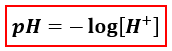# Problem: Calculate the pH of a 0.080 M carbonic acid solution, H 2CO3(aq), that has the stepwise dissociation constants Ka1 = 4.3 × 10 -7 and Ka2 = 5.6 × 10 -11.A) 1.10B) 3.73C) 6.37D) 10.25

🤓 Based on our data, we think this question is relevant for Professor Dixon's class at UCF.

###### FREE Expert Solution

We’re being asked to calculate the pH of a 0.080 M carbonic acid (H2CO3). Carbonic acid is a diprotic acid, it can donate two protons (H+and it will have two equilibrium reactions

To calculate for pH, we use the following equation:Given:

Ka1 = 4.3x10-7

1st equilibrium reaction:                H2CO3(aq) + H2O(l) → HCO3­-(aq)H3O+(aq

Ka2 = 5.6x10-11

2nd equilibrium reaction:               HCO3-(aq) + H2O(l) → CO32­-(aq)H3O+(aq)

H3O+(aq) is also produced from the 2nd equilibrium reaction###### Problem Details

Calculate the pH of a 0.080 M carbonic acid solution, H 2CO3(aq), that has the stepwise dissociation constants Ka1 = 4.3 × 10 -7 and Ka2 = 5.6 × 10 -11.

A) 1.10

B) 3.73

C) 6.37

D) 10.25

What scientific concept do you need to know in order to solve this problem?

Our tutors have indicated that to solve this problem you will need to apply the Diprotic Acid concept. You can view video lessons to learn Diprotic Acid. Or if you need more Diprotic Acid practice, you can also practice Diprotic Acid practice problems.

What is the difficulty of this problem?

Our tutors rated the difficulty ofCalculate the pH of a 0.080 M carbonic acid solution, H 2CO3...as high difficulty.

How long does this problem take to solve?

Our expert Chemistry tutor, Dasha took 6 minutes and 31 seconds to solve this problem. You can follow their steps in the video explanation above.

What professor is this problem relevant for?

Based on our data, we think this problem is relevant for Professor Dixon's class at UCF.